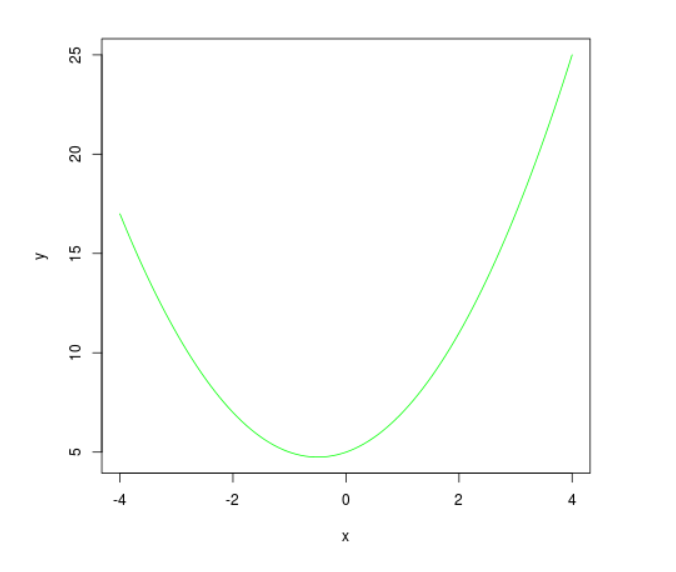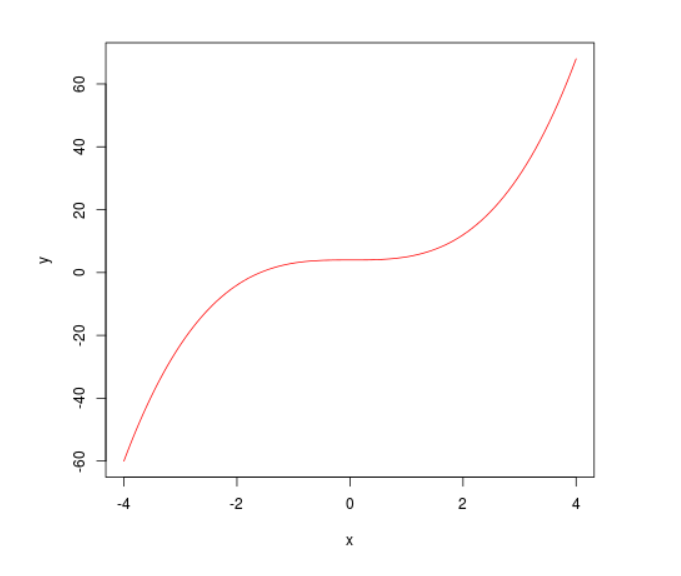GeeksforGeeks App
Open AppBrowser
Continue

# Create Line Curves for Specified Equations in R Programming – curve() Function

`curve()` function in R Language is used to draw a curve for the equation specified in the argument.

Syntax: curve(expression, to, from, col)

Parameters:
expression: To be curved
to, from: range of curve plotting
col: color of curve

Example 1:

 `# R program to create a curve`` ` `# Creating curve using curve() function``curve(x ^ ``2` `+` `x ``+` `5``, ``-``4``, ``4``, col ``=``"green"``, ylab ``=``"y"``)`

Output:Example 2:

 `# R program to create a curve`` ` `# Creating curve using function``fun <``-` `function(x) {x ^ ``3` `+` `4``}``curve(fun, ``-``4``, ``4``, col ``=``"red"``, ylab ``=``"y"``)`

Output:My Personal Notes arrow_drop_up